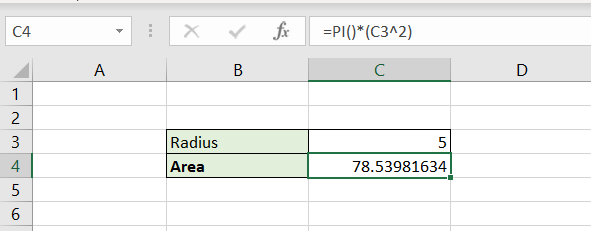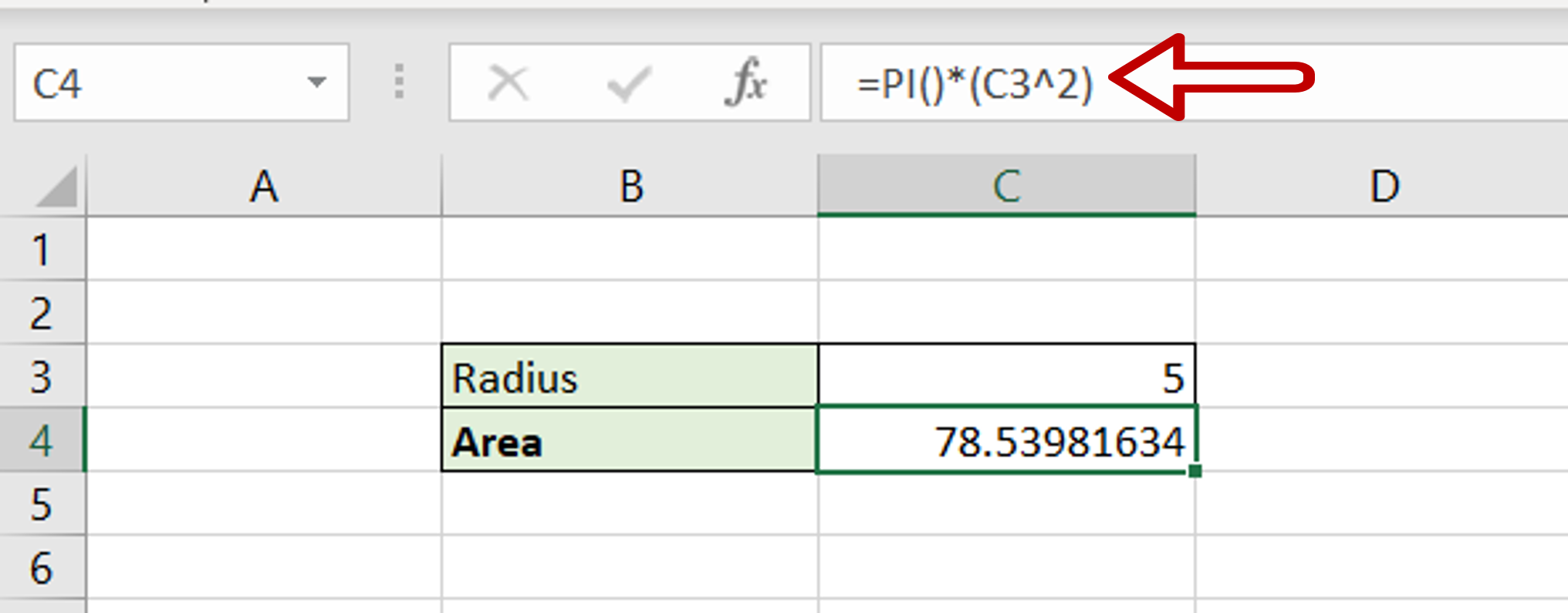# How to enter Pi in Excel

You can watch a video tutorial here.Excel is primarily used for its spreadsheet functions and has several functions to help with mathematical operations. One such function is the PI() function which inserts the value of Pi. Suppose you need to find the area of a circle, the formula for which is R2. You can do so by using the PI() function.

### Step 1 – Create the formula– Select the cell where the result is to appear
– Type the formula: